# Kerala Syllabus 9th Standard Physics Solutions Chapter 6 Current Electricity

You can Download Current Electricity Questions and Answers, Summary, Activity, Notes, Kerala Syllabus 9th Standard Physics Solutions Part 2 Chapter 6 help you to revise complete Syllabus and score more marks in your examinations.

## Kerala State State Syllabus 9th Standard Physics Solutions Chapter 6 Current Electricity

### Current Electricity Textual Questions and Answers

Current Electricity Class 9 Kerala Syllabus Chapter 6 Activity -1

A positively charged electroscope is connected to the earth through a switch using a conductor.9th Class Physics Chapter 6 Notes Kerala Syllabus Question 1.
What kind of charge is present in this electroscope?
StaticCurrent Electricity Class 9 Solutions Kerala Syllabus Chapter 6 Question 2.
What happens to this charge when the switch is turned on?
Charge neutralizes

9th Std Physics Notes Kerala Syllabus Chapter 6 Question 3.
Will the flow of charge sustain in this arrangement?
The charge will not sustain in this arrangement

9th Standard Physics Notes Kerala Syllabus Chapter 6 Activity – 2

A circuit with a cell, a bulb, and a switch is given in the figure.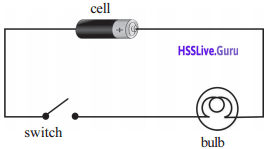Kerala Syllabus 9th Standard Physics Notes Chapter 6 Question 4.
Will the flow of electric current sustain in the circuit if it is switched on?
Yes, the flow of electric charge will sustain.

Current Electricity 9th Class Exercise Kerala Syllabus Chapter 6 Question 5.
What difference is there in the flow of current in both circuits?
In the first circuit, there is a flow of charge for a short interval of time. There is a continuous flow of charge in the second.

Kerala Syllabus 9th Standard Physics Notes Pdf Chapter 6 Question 6.
Complete the table based on different situations as shown in figure.Situation Direction of flow/motion Ball falling down Downwards from a higher level to a lower level Flow of air From a region of high pressure to a region of low pressure Flow of water From a higher level to lower level Flow of heat From a point having higher temperature to that having lower temperature

There should be a difference in energy levels between two points if any type of flow is to occur.

Kerala Syllabus 9th Standard Physics Notes Malayalam Medium Activity-3

observe the figuresQuestion 7.
If the value is opened, in which one will there be a flow of water and rotation of the wheel?Question 8.
Why?
It is due to the gravitational potential difference that there is a flow of water and consequent rotation of the wheel.

Activity-4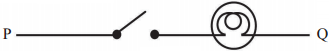A bulb is connected to a switch, using a conductor.

Question 9.
Will the bulb glow if switched on? Why?
The bulb will not glow
There is no potential difference between P and Q. Hence there is no flow of current and the bulb does not flow.Potential Difference and Current:
There should be a potential difference between two points of a conductor if there is to be flow of current between them. Current flows from a point of high electric potential to a point of low electric potential.

The unit of potential difference is volt (V). Voltameter is the device to measure this. If 1 joule of work is done to move one-coulomb charge from one point to another, then the potential difference between the points is 1 volt.

Activity – 5The pump has been used in such a way that the some quantity of water that flows from A to B per second is returned to Afrom B in the same period of time.

Question 10.
Why is there a continuous flow of water when the value is opened?
Here, it is due to the working of the pump, which is an external source of power, that the potential difference was maintained and the flow of water was made possible continuously.

Source of emf

An external source is needed to maintain a potential difference between the ends of a conductor and to maintain the flow of electric current through the conductor. That external source is called source of emf.
Eg: Generator, cell, battery, solar cell ………

Question 11.
Write down the energy change in each.
Generator: Mechanical energy → electrical energy
Cell (While discharging): Chemical energy → electrical energy
Battery (While discharging): Chemical energy → electrical energy
Solar cell: Solar energy → electrical energy

Question 12.
Complete the table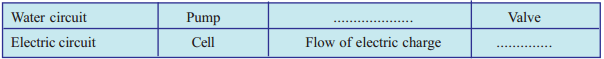Water circuit Pump Water wheel flow of water Value Electric circuit Cell bulb flow of electric charge Switch

A source of emf is essential to maintain a potential difference between the ends of a conductor and to maintain the flow of current through the conductor.

Activity – 6

Make a circuit given in figure using a voltmeter a 12V, 3W bulb, a cell, and a switch operate itQuestion 13.
How should-you connect a voltmeter in a circuit?
The voltmeter should be connected across the points (parallel) where the potential difference is to be mea¬sured.

Question 14.
In what mode are the cells connected within the remote control of a TV?
In series mode

Question 15.
If 4 cells of 1.5 V each are connected in series what is the total voltage?
4 × 1.5 = 6V

Question 16.
How can you connect four cells of 1.5V each to get 3V? What is the advantage of doing so?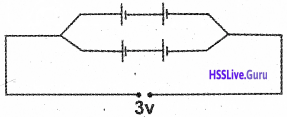If connected in this mode, we get electric current for a long time without variation in voltage.
Combination of cells:
A battery is a combination of two or more cells connected in a suitable manner. Cells can be connected in two ways.
1. Series connectionThis is the method of connecting cells one after the other in such a way that the positive of one cell is connected to the negative of another cell.
Salient features:

• The total emf is the sum of the emf of all the cells.
• The current passing through each cell is the same.
• The internal resistance developed in the circuit by the battery increases.
• The current in the external circuit increases under high voltage.

2. Parallel connectionThis is the method of connecting similar potes together.
Salient features:

• If all the cells have equal emf then the emf of the circuit is the same as that of a single cell.
• The total current flowing in the circuit splits up and flows through each cell.
• The internal resistance of the circuit is very low.
• More current can be made available for a longer time under low voltage.

Electric Current:
Electric current is the flow of electric charges. Current is the quantity of charge that flows through a conductor in a circuit in one second.

Question 17.
If 10 coulomb charge flows in a circuit in 5s, how much is the charge flowing in the circuit in one second?
Charge, Q = 10C
Time,t = 5s
Charge flowing in one second = $$\frac { 10 }{ 5 }$$ = 2 C/s

Question 18.
If a charge of Q coulomb flows in a time t second, then how much is the quality of charge that flows in one second?
Current (I) = $$\frac{\text { Quantity of charge }}{\text { Time taken }}$$
i.e I = Q/t

Question 19.
What is the unit of current?
Unit of current = $$\frac{\text { Unit of charge }}{\text { Unit of time }}$$
= C/s OR A

Activity -1

Make a circuit containing an ammeter, switch, cell and a bulb connected in series.Repeat the experiment by increasing the number of cells in series.

Question 20.
What change occurred in the ammeter reading when the number of cells was increased?

Question 21.
What about the intensity of light from the bulb?
Intensity of light increases

Question 22.
How are the current and the intensity of light related to each other?
As current in circuit increases, intensity of light increases.

Question 23.
What is the current in a conductor if 2 C charge flows in 10s?
$$\mathrm{I}=\frac{\mathrm{Q}}{\mathrm{t}}=\frac{2 \mathrm{c}}{10 \mathrm{s}}=0.2 \mathrm{C} / \mathrm{s}$$
= 0.2 A

AmmeterAmmeter is a device used to measure the current in a circuit. The positive terminal of it must be con¬nected directly to the positive of the cell and the negative terminal, to the negative of the cell. Ammeter should be connected in series in the circuit The needle of the device moves in accordance with the current in the circuit We can measure the current by checking the position of the needle. Unit of current is ampere (A), it is also written as C/s. mA(milliampere) and µA(microampere) are smaller units of current The symbol of ammeter isOhm’S Law

Make a circuit by including a nichrome wire (30cm), cell, switch, ammeter, and voltmeter.
Measure current (I) and potential difference (V). Repeat the activity by increasing the number of cells in series.Analyze the table and record the findings

Question 24.
What change occurred in the circuit when there was a change in voltage?
As voltage increases, current increases.

Question 25.
Do you see any peculiarity in the value of V/l? v
V/I will be a constant
V ∝ I
V = a constant × I
V/I = a constant
This constant is the resistance of the conductor. This is indicated by the letter R.
∴R = V/I
Ohm’s law:
When temperature remains constant, the current through a conductor is directly proportional to the potential difference between its ends. In other words, the ratio of potential difference to the current is a constant.
Resistors are conductors used to include a particular resistance in a circuit its symbol isQuestion 26.
On the basis of the information gathered from Table 6.5, draw a V-l graph, Mark I in the X – axis and V in the Y – axis.Question 27.
Is the graph a straight line?
Yes, the graph is a

Question 28.
What is the unit of resistance?
Unit of resistance = $$=\frac{\text { Unit of voltage }}{\text { Unit of Current }}$$
$$\frac{\text { Volt }}{\text { Ampere }}$$ or ohm (Ω )Question 29.
1 Ω = 1V/1A From this what do you mean by 1 ohm?
If the potential difference between the ends of a conductor is 1V when a current of 1A flows through it, then the resistance of the conductor is 1Ω.

When the potential difference between the ends of conductor is 1 Vand if a current of 1A flows through it, then the resistance of the conductor is 1Ω.
Using the given figure, from equation representing Ohm’s Law.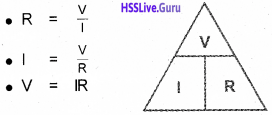Question 30.
Complete the following table based on Ohm’s Law

 Voltage (Volt V) Current (1)  ampere (A) Resistance (R)  ohm(Q) 12 …………………. 4 ………………….. 2 3 6 3 …………………..

 Voltage (Volt V) Current (I) ampere (A) Resistance (R) ohm(Q) 12 …… 3 …… 4 …… 6 ….. 2 3 6 3 ……. 2 ……..

Resistors

Arrange a circuit as shown in the figureAmong the conductors fixed on the wooden plank, PA is iron, PB is aluminum, PC, PD and PE are nichrome. Their lengths are the same. PE has double the length. The thickness of PD is double that of the others. Touch the free end J at A, B, C, D and record in the table the ammeter reading at each distance.

Question 31.
Is the intensity of light from the bulb the same in each situation?
No

Question 32.
Is the ammeter reading the same when different conductors of the same length and thickness were included?
No. The ammeter reading was not the same

Question 33.
What change has occurred in the ammeter reading when the area of cross-section of the same conductor is altered?
When the area of cross-section increases ammeter reading also increases.

Question 34.
Is there a change in the ammeter reading when the length of the same conductor is altered? Record,
Ammeter reading decreases with increase in length.

Question 35.
Is the applied potential difference the same in all cases?
Yes. The potential difference applied is the same.

Question 36.
According to Ohm’s Law, V/I must be a constant (resistance, R). If so, what is the reason for the changes in the ammeter readings?
The reason for the change in the ammeter reading is the variation of resistance of the conductors included in the circuit.

Activity -1

Connect a 6V bulb to a 6V source. Using a multimeter, measure the resistance of the bulb when the circuit is switched off. Switch on the bulb for a short time, then switch it off and immediately measure its resistance.

Question 37.
Is the resistance the same in both the situations?
No

Question 38.
When the circuit was switched on, was the temperature of the bulb low or high?
High

Question 39.
Did the resistance increase or decrease when the temperature was increased?
Resistance increased when the temperature was increased.

Question 40.
List the factors affecting the resistance of a conductor?

• Area of cross-section
• Nature of the material
• Length
• Temperature

Activity-2

In the activity conducted above, touch the free end J at E and slowly slide it from E to P

Question 41.
What change occurred in the intensity of light from the bulb?

Question 42.
What may be the reason behind the change?
As the length of the conductor decreases, resistance and current increases.

Question 43.
What is the working principle of a rheostat?
If the potential difference is constant, then the current is inversely proportional to the resistance. For a conductor of uniform area of cross-section, the length of a conductor and the resistance are directly proportional.

Rheostat is a device used to regulate the current in a circuit by changing the resistance

Question 44.
What is the symbol of a rheostat?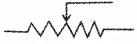Question 45.
Given below is a table related to the resistance of a conductor. Complete the table suitably.

Question 46.
Analysis the completed table and write down the inferences.
The resistance of a conductor increases with the increases in the length of the conductor.
R α l
The resistance of a conductor decreases with increases in the area of cross-section.
R α I/A that is R α I/A
R = a constant × l/A
R = $$\rho \frac{1}{\mathrm{A}}$$
$$\rho$$ = RA/l
P is the resistivity of the material the conductor is made of. The length of a conductor of resistance R Q is 1m and its area of cross-section is 1m2. Calculate the resistivity of the material the conductor is made of. length, l= 1m
Area of cross-section, A = 1m2
Resistivity $$\rho=\frac{R A}{1}=\frac{R \times 1}{1} \quad \rho=R$$
Resistivity of a substance is the resistance of the conductor of unit length and unit area of cross-section. The resistivity of a substance is a constant at fixed temperature. But it will be different for different materials.
Unit of resistivity =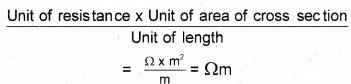The Unit of resistivity is Qm
Let’s get acquainted with some of the tools related to electric current:
We use many electric devices in our everyday life. Different tools are needed to connect these devices with the electric line and to perform maintenance. They are enlisted here.

Screwdriverit helps in fixing and removing the screws Screwdrivers are available in different sizes It is used to combine a wide range of screws with +,* shaped edges.

Electric testerIt is used to check whether current is coming into the sockets or other devices in the houses Some of these can be used as screwdriver. The bulb in¬side the tester will glow if there is presence of current.

Wire stripperIt is used to remove insulation of wires while combining insulated electric wires or when they are to be connected to the devices

PliersIt is used to join wires by twisting them together or for cutting or removing wires. Pliers are available in different shapes and sizes

Gloves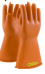While doing the work related to electric power, gloves are worn in the hand as a protection from electric shock.

Multimeter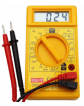It is used to measure current, voltage, and resistance in a circuit and to understand whether the circuit is open, closed or any connection is left. Besides, it also helps to check whether the various elements in an electronic circuit are functioning properly.

Clamp ammeter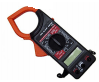It helps to measure the current in a circuit without connecting wires or devices in the circuit.

Insulation tapeWhen connecting the wires or connecting it with a device, this is used to provide insulation in those parts where it has been damaged.

Spanner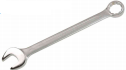It is used for fixing nut and bolt. They are available in different sizes.

Soldering iron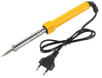It is used to solder electronic components in a circuit

Hammer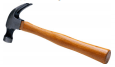It is used for fixing and removing nails.

Drill machine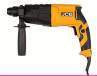It is used to drill holes on hard surfaces. It can be used to fix and remove screws as well.

Let Us Assess

Question 1.
complete the table properly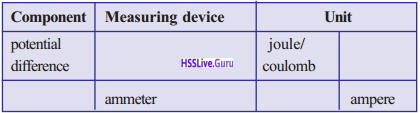Question 2.
Given below are the diagrams showing the connection of ammeter and voltmeter in a circuit. Of these, which are correct?Question 3.
Complete the table. The conductor is made of the same material.

 Length of conductor Area of cross-section of conductor Resistance of the conductor 1 cm 2 cm2 10 Q 2 cm 2cm2 20 Q 1 cm 4cm2 5Q

Question 4.
In an electrical circuit if 100 J work is done to move 10 C electric charge from point A to the point B, find out the potential difference between the points A& B.
100/10 = 10V

Question 5.
6 electric cells are connected in series in an elec¬tronic device which works at 9 V potential difference. Find out emf of one cell.
9/6 = 1.5VQuestion 6.
An ammeter that connects to an electronic circuit shows a reading of 2A. Find how many charges flows through the ammeter in 10 s.
Q = I x t = 2 x 10 = 20 coulomb

Question 7.
When a conductor is stretched, its length becomes double. Find out how many times the resistance changes.
4 times.

Question 8.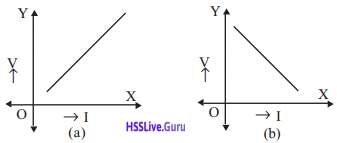In the given graphs which is the graph depicting Ohm’s Law? Justify your answer.
(a) v ∝ I, As V increases, I also increase.

Question 9.
A conductor of 5 Q resistance has length 2m and area of cross-section 2 m2. If so, find out the resistivity of the material of the conductor.Question 10.
Draw a circuit diagram describing how 6 torch cells should be connected to a bulb and a switch to obtain effective voltage of 9 V.### Current Electricity More Questions

Question 1.
The resistance of a 10cm long wire is 120. If this is folded into two parts of equal length and included in a circuit, how much will be the resistance produced?
When folded into two parts, length is halved and area of cross-section is doubled. Due to the decrease in length, resistance is halved. Also due to the decrease in area of cross-section, resistance is again halved.

So effective resistance R = $$12 \times \frac{1}{2} \times \frac{1}{2}=3 \Omega$$

Question 2.
Of the following, which one correctly indicates Ohm’s Law?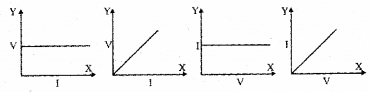I = $$\frac { V }{ R }$$ = $$\frac { 6 }{ 12 }$$ = 0.5A
I = $$\frac { V }{ R }$$ = $$\frac { 12 }{ 6 }$$ = 2A